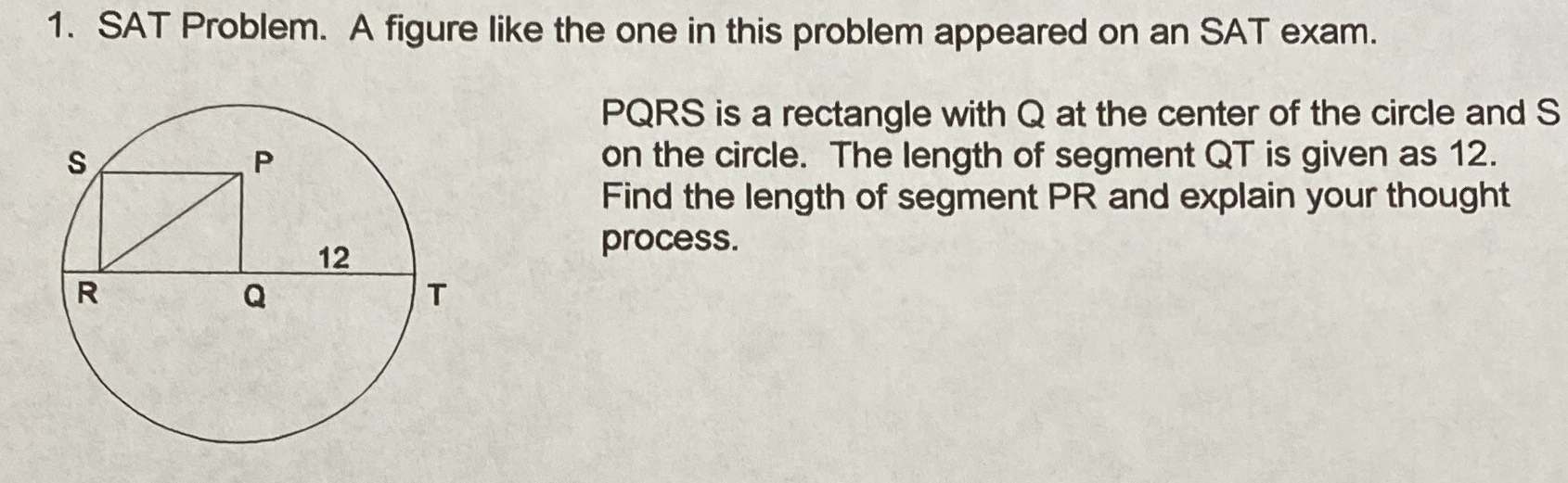### ¿Todavía tienes preguntas de matemáticas?

Pregunte a nuestros tutores expertos
Algebra
PreguntaSAT Problem. A figure like the one in this problem appeared on an SAT exam.

PQRS is a rectangle with $$Q$$ at the center of the circle and $$S$$ on the circle. The length of segment QT is given as $$12$$ . Find the length of segment $$P R$$ and explain your thought process.

$$12$$